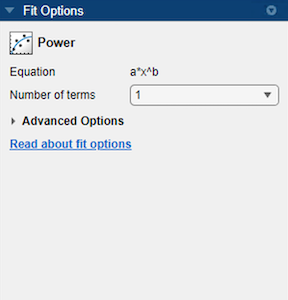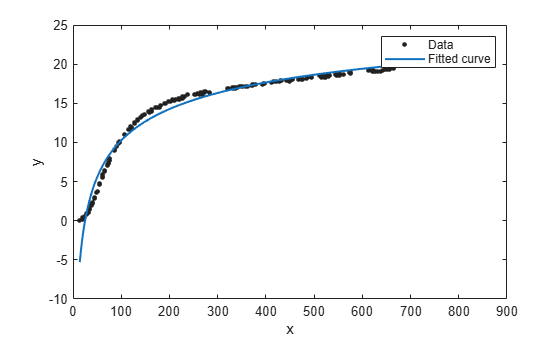## Power Series

The toolbox provides a one-term and a two-term power series model as given by

`$\begin{array}{l}y=a{x}^{b}\\ y=a{x}^{b}+c\end{array}$`

Power series models describe a variety of data. For example, the rate at which reactants are consumed in a chemical reaction is generally proportional to the concentration of the reactant raised to some power.

### Fit Power Series Models Interactively

1. Open the Curve Fitting app by entering `cftool`. Alternatively, click Curve Fitting on the Apps tab.

2. In the Curve Fitting app, select curve data (X data and Y data, or just Y data against index).

Curve Fitting app creates the default curve fit, `Polynomial`.

3. Change the model type from `Polynomial` to `Power`.You can specify the following options:

• Choose the number of terms: `1` to `2`.

Look in the Results pane to see the model terms, the values of the coefficients, and the goodness-of-fit statistics.

• (Optional) Click Fit Options to specify coefficient starting values and constraint bounds, or change algorithm settings.

The toolbox calculates optimized start points for power series models, based on the current data set. You can override the start points and specify your own values in the Fit Options dialog box.

For more information on the settings, see Specifying Fit Options and Optimized Starting Points.

### Fit Power Series Models Using the fit Function

This example shows how to use the `fit` function to fit power series models to data.

The power series library model is an input argument to the `fit` and `fittype` functions. Specify the model type `'power1'` or `'power2'` .

Fit a Single-Term Power Series Model

```load hahn1; f = fit(temp,thermex,'power1')```
```f = General model Power1: f(x) = a*x^b Coefficients (with 95% confidence bounds): a = 1.46 (1.224, 1.695) b = 0.4094 (0.3825, 0.4363) ```
`plot(f,temp,thermex)`Fit a Two-Term Power Series Model

`f = fit(temp,thermex,'power2')`
```f = General model Power2: f(x) = a*x^b+c Coefficients (with 95% confidence bounds): a = -78.61 (-80.74, -76.48) b = -0.2349 (-0.271, -0.1989) c = 36.9 (33.09, 40.71) ```
`plot(f,temp,thermex)`